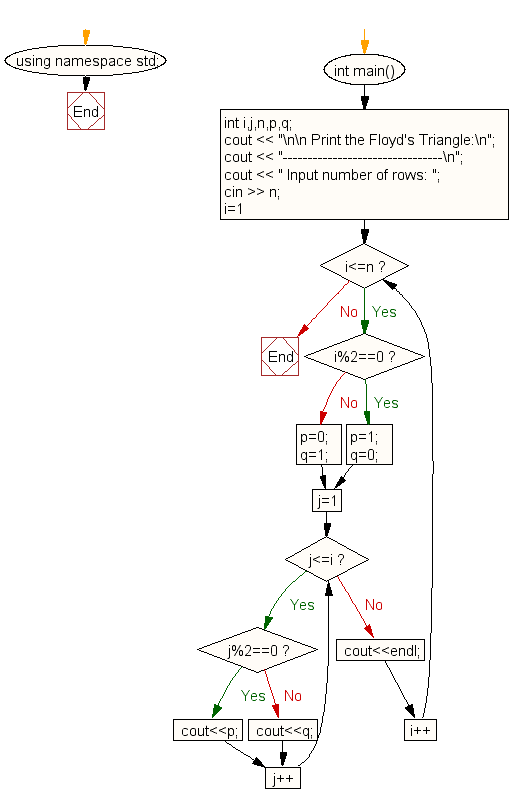﻿ C++ Exercises: Print the Floyd's Triangle - w3resource

# C++ Exercises: Print the Floyd's Triangle

## C++ For Loop: Exercise-43 with Solution

Write a C++ program to print Floyd's Triangle.

Sample Solution:

C++ Code :

``````#include <iostream>
using namespace std;

int main()
{
int i,j,n,p,q;
cout << "\n\n Print the Floyd's Triangle:\n";
cout << "--------------------------------\n";
cout << " Input number of rows: ";
cin >> n;
for(i=1;i<=n;i++)
{
if(i%2==0)
{
p=1;q=0;
}
else
{
p=0;q=1;
}
for(j=1;j<=i;j++)
if(j%2==0)
cout<<p;
else
cout<<q;
cout<<endl;
}
}
``````

Sample Output:

``` Print the Floyd's Triangle:
--------------------------------
Input number of rows: 5
1
01
101
0101
10101
```

Flowchart:C++ Code Editor: